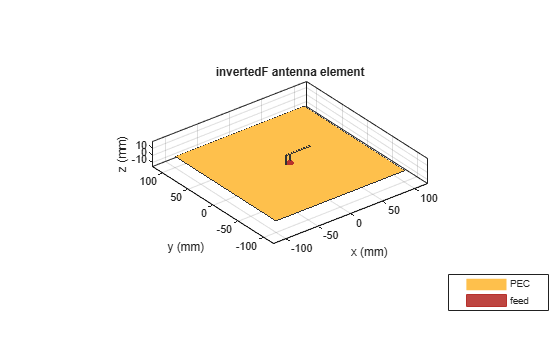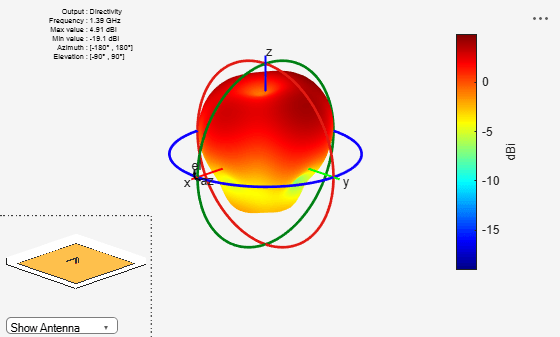invertedF

Create inverted-F antenna over rectangular ground plane

Description

The invertedF object is an inverted-F antenna mounted over a rectangular ground plane.

The width of the metal strip is related to the diameter of an equivalent cylinder by the equation

$w=2d=4r$

where:

• d is the diameter of equivalent cylinder

• r is the radius of equivalent cylinder

For a given cylinder radius, use the utility function cylinder2strip to calculate the equivalent width. The default inverted-F antenna is center-fed. The feed point coincides with the origin. The origin is located on the xy- plane.Creation

Description

f = invertedF creates an inverted-F antenna mounted over a rectangular ground plane. By default, the dimensions are chosen for an operating frequency of 1.7 GHz.

example

f = invertedF(Name,Value) creates an inverted-F antenna, with additional properties specified by one, or more name-value pair arguments. Name is the property name and Value is the corresponding value. You can specify several name-value pair arguments in any order as Name1, Value1, ..., NameN, ValueN. Properties not specified retain their default values.

Properties

expand all

Vertical element height along z-axis, specified a scalar in meters.

Example: 'Height',3

Data Types: double

Strip width, specified as a scalar in meters.

Note

Strip width should be less than 'Height'/4 and greater than 'Height'/1001. 

Example: 'Width',0.05

Data Types: double

Stub length from feed to open end, specified as a scalar in meters.

Example: 'LengthToOpenEnd',0.05

Stub length from feed to shorting end, specified as a scalar in meters.

Example: 'LengthToShortEnd',0.0050

Ground plane length along x-axis, specified as a scalar in meters. Setting 'GroundPlaneLength' to Inf, uses the infinite ground plane technique for antenna analysis.

Example: 'GroundPlaneLength',4

Data Types: double

Ground plane width along y-axis, specified as a scalar in meters. Setting 'GroundPlaneWidth' to Inf, uses the infinite ground plane technique for antenna analysis.

Example: 'GroundPlaneWidth',2.5

Data Types: double

Signed distance from center along length and width of ground plane, specified as a two-element vector.

Example: 'FeedOffset',[2 1]

Data Types: double

Type of the metal used as a conductor, specified as a metal material object. You can choose any metal from the MetalCatalog or specify a metal of your choice. For more information, see metal. For more information on metal conductor meshing, see Meshing.

Example: m = metal('Copper'); 'Conductor',m

Example: m = metal('Copper'); ant.Conductor = m

Lumped elements added to the antenna feed, specified as a lumped element object. For more information, see lumpedElement.

Example: 'Load',lumpedelement. lumpedelement is the object for the load created using lumpedElement.

Tilt angle of the antenna, specified as a scalar or vector with each element unit in degrees. For more information, see Rotate Antennas and Arrays.

Example: 'Tilt',90

Example: ant.Tilt = 90

Example: 'Tilt',[90 90],'TiltAxis',[0 1 0;0 1 1] tilts the antenna at 90 degrees about the two axes defined by the vectors.

Note

The wireStack antenna object only accepts the dot method to change its properties.

Data Types: double

Tilt axis of the antenna, specified as:

• Three-element vector of Cartesian coordinates in meters. In this case, each coordinate in the vector starts at the origin and lies along the specified points on the X-, Y-, and Z-axes.

• Two points in space, each specified as three-element vectors of Cartesian coordinates. In this case, the antenna rotates around the line joining the two points in space.

• A string input describing simple rotations around one of the principal axes, 'X', 'Y', or 'Z'.

Example: 'TiltAxis',[0 1 0]

Example: 'TiltAxis',[0 0 0;0 1 0]

Example: ant.TiltAxis = 'Z'

Note

The wireStack antenna object only accepts the dot method to change its properties.

Data Types: double

Object Functions

 show Display antenna or array structure; display shape as filled patch info Display information about antenna or array axialRatio Axial ratio of antenna beamwidth Beamwidth of antenna charge Charge distribution on metal or dielectric antenna or array surface current Current distribution on metal or dielectric antenna or array surface design Design prototype antenna or arrays for resonance around specified frequency efficiency Radiation efficiency of antenna EHfields Electric and magnetic fields of antennas; Embedded electric and magnetic fields of antenna element in arrays impedance Input impedance of antenna; scan impedance of array mesh Mesh properties of metal or dielectric antenna or array structure meshconfig Change mesh mode of antenna structure optimize Optimize antenna or array using SADEA optimizer pattern Radiation pattern and phase of antenna or array; Embedded pattern of antenna element in array patternAzimuth Azimuth pattern of antenna or array patternElevation Elevation pattern of antenna or array rcs Calculate and plot radar cross section (RCS) of platform, antenna, or array returnLoss Return loss of antenna; scan return loss of array sparameters Calculate S-parameter for antenna and antenna array objects vswr Voltage standing wave ratio of antenna

Examples

collapse all

Create and view an inverted-F antenna with 14 mm height over a ground plane of dimensions 200 mm-by-200 mm.

f = invertedF('Height',14e-3, 'GroundPlaneLength',200e-3,          ...
'GroundPlaneWidth',200e-3);
show(f)This example shows you how to plot the radiation pattern of an inverted-F antenna for a frequency of 1.3 GHz.

f = invertedF('Height',14e-3, 'GroundPlaneLength', 200e-3,          ...
'GroundPlaneWidth', 200e-3);
pattern(f,1.39e9)Balanis, C.A. Antenna Theory. Analysis and Design, 3rd Ed. New York: Wiley, 2005.

 Volakis, John. Antenna Engineering Handbook, 4th Ed. New York: Mcgraw-Hill, 2007.